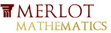# Effect of Coefficient of x^2 on Parabola Vertex (a < 0)This material was originally developed through Merlot
as part of its collaboration with the SERC Pedagogic Service.

#### Summary

This classroom activity presents College Algebra students with a ConcepTest, a Question of the Day, and a Write-pair-share activity concerning the effect of the coefficient of x^2 on the vertex of a parabola where a<0, b>0 and b and c are arbitrarily fixed values in f(x)=ax^2+bx+c.

The results are quite revealing and show that while students may have learned that the coefficient of x^2 affects the shape of a parabola, most do not realize that it also affects the position of the vertex when b is not equal to 0. Algebraically analyzing the effects requires a good bit of effort on the students' part, but the results are rewarding.

## Learning Goals

To enable students to:

• develop their understanding of the effects of coefficients in quadratic functions
• exercise their mathematical intuition and verify it via appropriate calculations
• exercise the skills of algebraic and mathematical analysis

## Context for Use

This activity can be carried out in either a small College Algebra class or a large lecture setting anytime during or after the topic of graphing quadratic functions has been covered.

The activity is comprised of four segments: ConcepTest, Question of the Day, Write-pair-share exercise, and conclusion involving the use of a demonstration applet. The time required for the entire activity is approximately 40 minutes but fewer segments can be offered as a shorter alternative (see Activity Description below for individual segment times).

## Description and Teaching Materials

Activity Description and Teaching Materials

• Instructor presents a ConcepTest (Rich Text File 75kB Aug18 10) in the form of a straw poll (either show-of-hands or written) concerning the effect of increasing the value of the coefficient of x^2 on the position of the vertex. Each student is asked to make a conjecture and the instructor records the results for the class to see. (~5 minutes)
• After the straw poll, the question becomes the Question of the Day (Rich Text File 72kB Aug18 10) and students work in pairs to share and explain their reasoning in written form. (~10 minutes)
• Lastly, students are asked to carry out the appropriate calculations in a write-pair-share activity (Rich Text File 136kB Aug18 10) to verify their conjectures. (~15 minutes)
• In conclusion, the instructor presents a classroom demo involving a Java applet that quickly and easily demonstrates the effects of changing the value of the coefficient of x^2 in a quadratic function. http://www.ltcconline.net/greenl/java/Other/MovingGraph/MovingGraph.html

In addition, the instructor ensures that students understand the algebraic and mathematical analysis involving the expanded form of the formula for the vertex by clearly explaining how the coefficient affects each of the x- and y-coordinates of the vertex. (~10 minutes)

## Teaching Notes and Tips

Student understanding of the role of coefficients in polynomial functions is limited at best. Most have not considered the vertex in terms of the values of a, b and c, and many have difficulty in analyzing the effects of changing the values of these coefficients. The fact that increasing the value of the denominator in a fraction actually lessens the value of the fraction as a whole sometimes seems to escape them. This activity is designed to help them develop a better grasp of the role of coefficients.

The concept of a limiting value for the vertex, (0,c), as a approaches negative infinity may also be addressed.

## Assessment

I generally give the students a grade for participation in this activity and sometimes grade their final calculations concerning the work required.

## References and Resources

Larry Green's Applet Page-Parameters and Graphs
This applet allows the user to change the values of the coefficients of a quadratic function and immediately see the graphical results.
http://www.ltcconline.net/greenl/java/index.html
http://www.ltcconline.net/greenl/java/Other/MovingGraph/MovingGraph.html
MERLOT description of this resource
http://www.merlot.org/merlot/viewMaterial.htm?id=77279